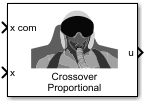# Crossover Pilot Model

Represent crossover pilot model

•Libraries:
Aerospace Blockset / Pilot Models

## Description

The Crossover Pilot Model block represents the pilot model described in Mathematical Models of Human Pilot Behavior ). This pilot model is a single input, single output (SISO) model that represents some aspects of human behavior when controlling aircraft.

The Crossover Pilot Model takes into account the combined dynamics of the human pilot and the aircraft, using the form described in Algorithms around the crossover frequency.

This block has nonlinear behavior. If you want to linearize the block (for example, with one of the `linmod` functions), you might need to change the Pade approximation order. The Crossover Pilot Model block implementation incorporates the Transport Delay block with the Pade order (for linearization) parameter set to `2` by default. To change this value, use the `set_param` function, for example:

`set_param(gcb,'pade','3')`

When modeling human pilot models, use this block for more accuracy than that provided by the Tustin Pilot Model block. This block is also less accurate than the Precision Pilot Model block.

## Ports

### Input

expand all

Signal command that the pilot model controls, specified as a scalar.

Data Types: `double`

Signal that the pilot model controls, specified as a scalar.

Data Types: `double`

### Output

expand all

Aircraft command, returned as a scalar.

Data Types: `double`

## Parameters

expand all

Dynamics control that you want the pilot to have over the aircraft. This table lists the options and associated dynamics.

Option (Controlled Element Transfer Function) Transfer Function of Controlled Element ( Y c ) Transfer Function of Pilot ( Y p ) Y c Y p Notes
```Proportion al```

`${K}_{c}$`

`$\frac{{K}_{p}{e}^{-\tau s}}{s}$`

`$\frac{{K}_{c}{K}_{p}{e}^{-\tau s}}{s}$`

`Rate or velocity`

`$\frac{{K}_{c}}{s}$`

`${K}_{p}{e}^{-\tau s}$`

`$\frac{{K}_{c}{K}_{p}{e}^{-\tau s}}{s}$`

`Spiral divergence`

`$\frac{{K}_{c}}{{T}_{I}s-1}$`

`${K}_{p}{e}^{-\tau s}$`

`$\frac{{K}_{c}{K}_{p}{e}^{-\tau s}}{\left({T}_{I}s-1\right)}$`

`Second order - Short period`

`$\frac{{K}_{c}{\omega }_{n}{}^{2}}{{s}^{2}+2\zeta {\omega }_{n}s+{\omega }_{n}^{2}}$`

`$\frac{{K}_{p}{e}^{-\tau s}}{{T}_{I}s+1}$`

`$\begin{array}{l}\frac{{K}_{c}{\omega }_{n}{}^{2}}{{s}^{2}+2\zeta {\omega }_{n}s+{\omega }_{n}^{2}}×\\ \frac{{K}_{p}{e}^{-\tau s}}{{T}_{I}s+1}\end{array}$`

Short
period,
with ${\omega }_{n}>1/\tau$
`Acceleration (*) `

`$\frac{{K}_{c}}{{s}^{2}}$`

`${K}_{p}s{e}^{-\tau s}$`

`$\frac{{K}_{c}{K}_{p}{e}^{-\tau s}}{s}$`

`Roll attitude (*)`

`$\frac{{K}_{c}}{s\left({T}_{I}s+1\right)}$`

`${K}_{p}\left({T}_{L}s+1\right){e}^{-\tau s}$`

`$\frac{{K}_{c}{K}_{p}{e}^{-\tau s}}{s}$`

With
T L ≈ T I
`Unstable short period(*)`

`$\frac{{K}_{c}}{\left({T}_{I1}s+1\right)\left({T}_{I2}s-1\right)}$`

`${K}_{p}\left({T}_{L}s+1\right){e}^{-\tau s}$`

`$\frac{{K}_{c}{K}_{p}{e}^{-\tau s}}{\left({T}_{I2}s-1\right)}$`

With
T L ≈ T I1
`Second order - Phugoid(*)`

`$\frac{{K}_{c}{\omega }_{n}{}^{2}}{{s}^{2}+2\zeta {\omega }_{n}s+{\omega }_{n}^{2}}$`

`${K}_{p}\left({T}_{L}s+1\right){e}^{-\tau s}$`

`$\frac{{K}_{c}{K}_{p}{\omega }_{n}^{2}{e}^{-\tau s}}{s}$`

Phugoid,
with $\begin{array}{l}{\omega }_{n}\ll 1/\tau ,\\ 1/{T}_{L}\approx \zeta {\omega }_{n}\end{array}$

* Indicates that the pilot model includes a Derivative block, which produces a numerical derivative. For this reason, do not send discontinuous (such as a step) or noisy input to the Crossover Pilot Model block. Such inputs can cause large outputs that might render the system unstable.

This table defines the variables used in the list of control options.

VariableDescription
K c Aircraft gain.
K p Pilot gain.
τPilot time delay.
T I Lag constant.
ζDamping ratio for the aircraft.
ω n Natural frequency of the aircraft.

#### Dependencies

The Crossover Pilot Model parameters are enabled and disabled according to the Type of control options. The Calculated value, Controlled element gain, Pilot gain, Crossover frequency (rad/s), and Pilot time delay(s) parameters are always enabled.

#### Programmatic Use

 Block Parameter: `sw_popup` Type: character vector Values: `'Proportion'` | ```'Rate or velocity'``` |`'Spiral divergence'` | `'Second order - Short period'` | `'Acceleration(*)'` | ```'Roll attitude(*)'``` | ```'Unstable short period(*)'``` | ```'Second order - Phugoid(*)'``` Default: `'Proportion'`

Crossover frequency or pilot gain value you want the block to calculate:

• `Crossover frequency` — The block calculates the crossover frequency value. The parameter value is disabled.

• `Pilot gain` — The block calculates the pilot gain value. The parameter value is disabled.

#### Programmatic Use

 Block Parameter: `freq_gain_popup` Type: character vector Values: ```'Crossover frequency'``` | `'Pilot gain'` Default: ```'Crossover frequency'```

Controlled element gain, specified as a double scalar.

#### Programmatic Use

 Block Parameter: `Kc` Type: character vector Values: double scalar Default: `'1'`

Pilot gain, specified as a double scalar.

#### Dependencies

To enable this parameter, set Calculated value to `Pilot gain`.

#### Programmatic Use

 Block Parameter: `Kp` Type: character vector Values: double scalar Default: `'3'`

Crossover frequency value, specified as double scalar, in rad/s. The value must be in the range between 1 and 10.

#### Dependencies

To enable this parameter, set Calculated value to `Crossover frequency`.

#### Programmatic Use

 Block Parameter: `omega_c` Type: character vector Values: double scalar Default: `'3'`

Total pilot time delay, specified as a double scalar, in seconds. This value typically ranges from 0.1 s to 0.2 s.

#### Programmatic Use

 Block Parameter: `time_delay` Type: character vector Values: double scalar Default: `'0.1'`

Pilot lead constant, specified as a double scalar.

#### Dependencies

To enable this parameter, set Type of control to one of the following options:

• `Roll attitude (*)`

• `Unstable short period (*)`

• `Second order - Phygoid(*)`

#### Programmatic Use

 Block Parameter: `T` Type: character vector Values: double scalar Default: `'1'`

Pilot lag constant, specified as a double scalar.

#### Dependencies

To enable this parameter, set Type of control to `Second order - Short period`.

#### Programmatic Use

 Block Parameter: `Ti` Type: character vector Values: double scalar Default: `'5'`

## Algorithms

The Crossover Model takes into account the combined dynamics of the human pilot and the aircraft, using the following form around the crossover frequency:

`${Y}_{p}{Y}_{c}=\frac{{\omega }_{c}{e}^{-\tau s}}{s},$`

Where:

VariableDescription
Y p Pilot transfer function.
Y c Aircraft transfer function.
ω c Crossover frequency.
τ Transport delay time caused by the pilot neuromuscular system.

If the dynamics of the aircraft (Yc) change, Yp changes correspondingly.

Note

This block is valid only around the crossover frequency. It is not valid for discrete inputs such as a step.

 McRuer, D. T., Krendel, E., Mathematical Models of Human Pilot Behavior. Advisory Group on Aerospace Research and Development AGARDograph 188, Jan. 1974.